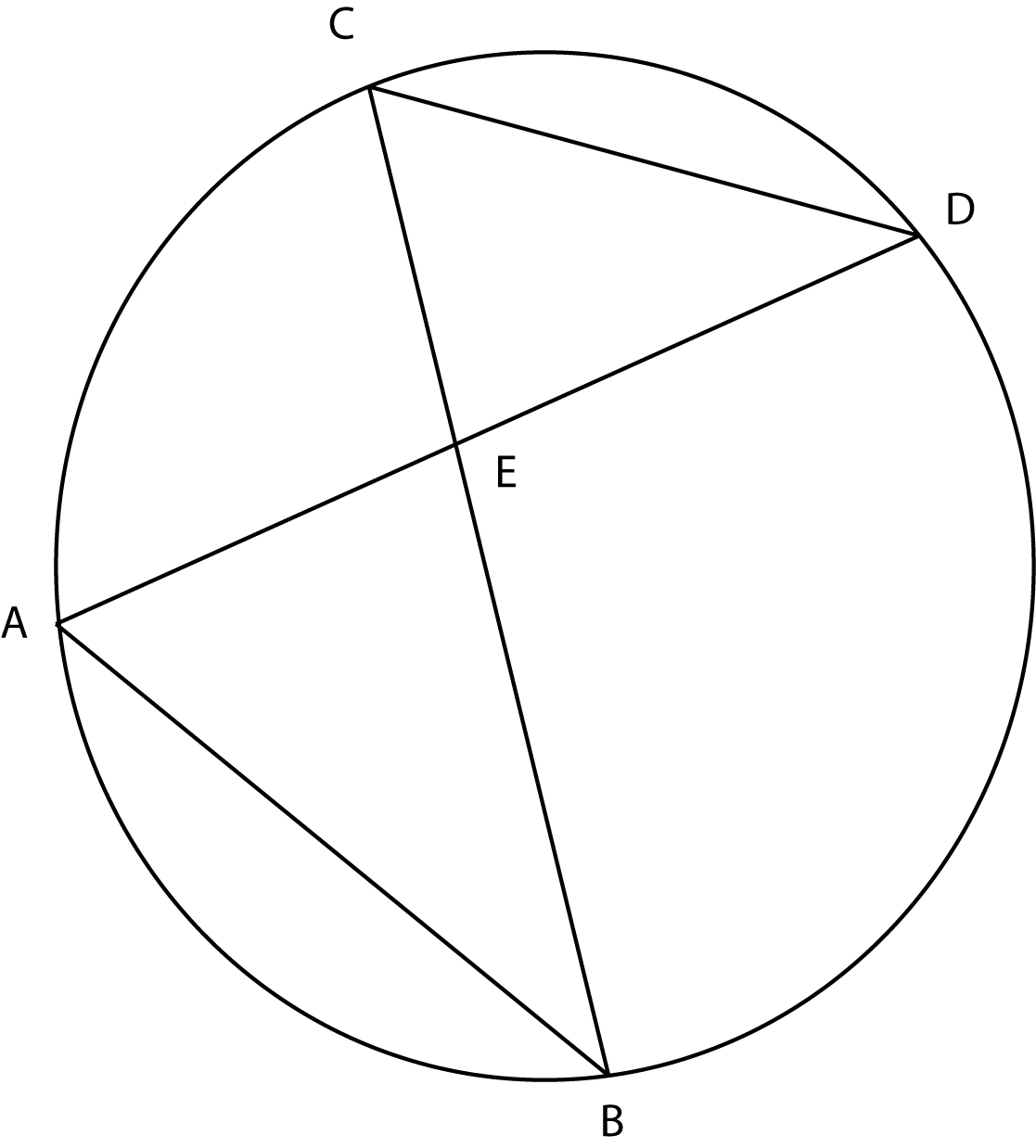### Prove: AE / CE = AB / CDAA similarity
<DAB=(1/2) * (arc DB) and <DCB=(1/2) * (arc DB)
<DAB =<DCB
AE / CE = AB / CD
Substitution
<AEB = <CED
triangle AEB ~ triangle CED
Inscribed angle measures half its intercepted arc
Definition
Vertical angles are equal